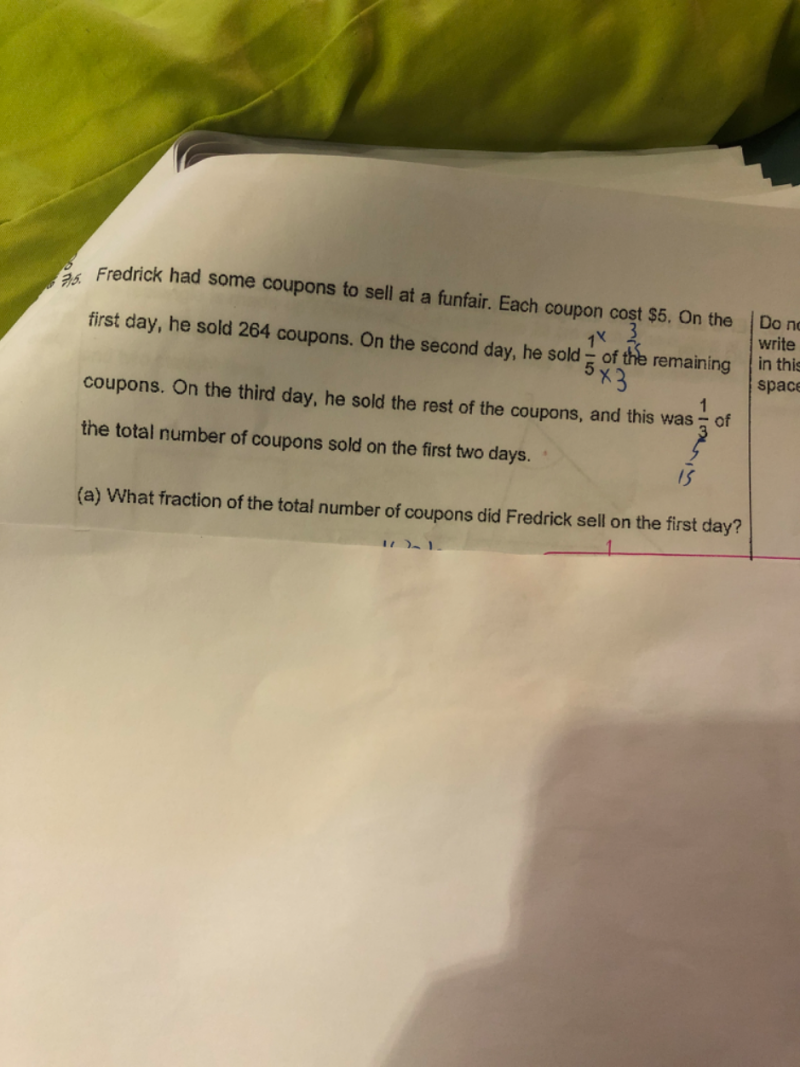# QuestionPls help. Thanks

(a) Let u be the total coupons.

1st day  sale       =  264

2nd day sale    = (1/5)(u-264)

3rd day sale  = (4/5)(u-264)

Given 3rd day sale =  (1/3)(1st + 2nd day sale)

(4/5)(u-264)   = (1/3) [ 264 + (1/5)(u-264) ]     (x 15 for both sides)

12u – 3168 = 1320 + u – 264

11u = 4224   =>  u = 384

Hence fraction of coupons sold on the 1st day = 264 / 384 = 11/16

0 Replies 2 Likes ✔Accepted Answer